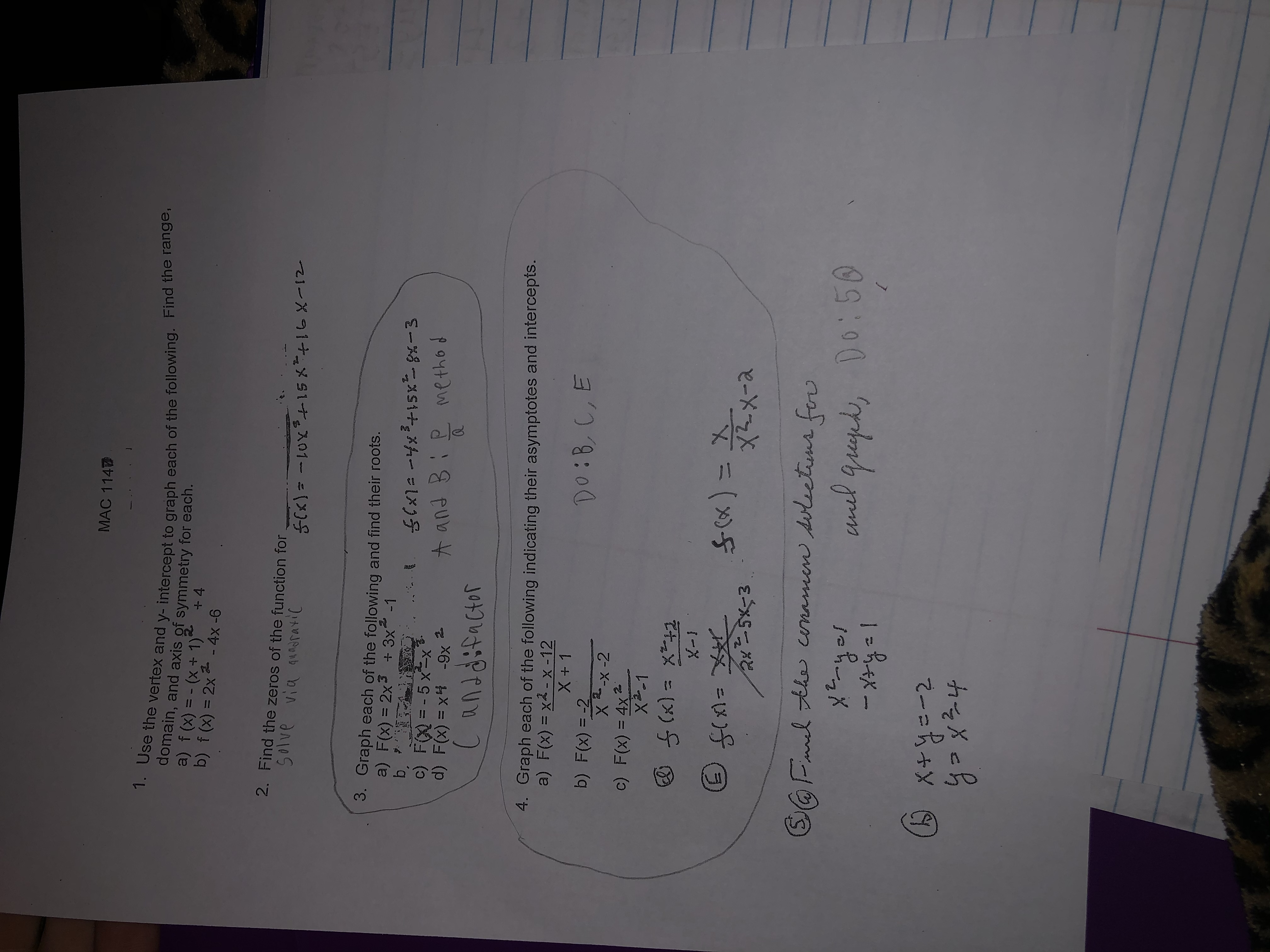# MAC 1141. Use the vertex and y- intercept to graph each of the following. Find the range,domain, and axis of symmetry for each.a) f (x) = - (x+ 1) +4b) f (x) = 2x 2 - 4x -6%3D2. Find the zeros of the function forSolve via quadratic3. Graph each of the following and find their roots.a) F(x) = 2x3 + 3x -1b,c) F() = - 5 x=xd) F(x) = x4 -9x 2.%3D..A and Bie methodCanddifactor4. Graph each of the following indicating their asymptotes and intercepts.a) F(x) = x2 - x -12Do:B,C,Eb) F(x) = -2X-x-2%Dc) F(x) = 4x2X2-1%3DX-XS(x) = X_X2.x-afix)=X2x5x=3%3DSOFmel the conamen soleetuns fovxE-yolunel Grupk, Do:50Comox+y=-2yoxz4

Question
12 views

How do you answer question 5?help_outlineImage TranscriptioncloseMAC 114 1. Use the vertex and y- intercept to graph each of the following. Find the range, domain, and axis of symmetry for each. a) f (x) = - (x+ 1) +4 b) f (x) = 2x 2 - 4x -6 %3D 2. Find the zeros of the function for Solve via quadratic 3. Graph each of the following and find their roots. a) F(x) = 2x3 + 3x -1 b, c) F() = - 5 x=x d) F(x) = x4 -9x 2. %3D .. A and Bie method Canddifactor 4. Graph each of the following indicating their asymptotes and intercepts. a) F(x) = x2 - x -12 Do:B,C,E b) F(x) = -2 X-x-2 %D c) F(x) = 4x2 X2-1 %3D X-X S(x) = X_ X2.x-a fix)=X 2x5x=3 %3D SOFmel the conamen soleetuns fov xE-yol unel Grupk, Do:50 Como x+y=-2 yoxz4 fullscreen
check_circle

Step 1
Step 2

Graph:

Step 3

### Want to see the full answer?

See Solution

#### Want to see this answer and more?

Solutions are written by subject experts who are available 24/7. Questions are typically answered within 1 hour.*

See Solution
*Response times may vary by subject and question.
Tagged in